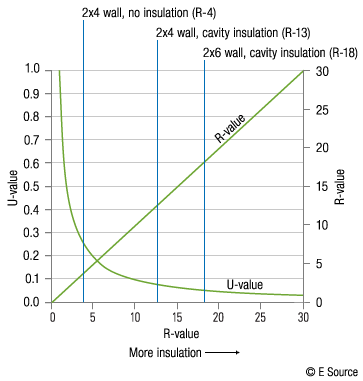# Thermal transmittance U vs th. resistance R

To achieve thermal comfort and energy efficiency in buildings, a primary role is played by the thermal envelope: this is required to thermally decouple the indoor environment from the ever-changing external conditions, both in summer and in winter.

The structures of the thermal envelope need to be able to control the amount of heat migrating through them: the thermal resistance and the thermal transmittance are two ways to describe this phenomenon.

Thermal Resistance and Th. Transmittance are two ways to describe how “insulated” a structure is: each of them has its pros and cons, but they are the two sides of the same coin.

The base assumption is to have a constant heat flow through an opaque structure, defined by two parallel flat surfaces. This is the case of external walls (or roof) of a building, in winter. The thermal behavior of the opaque components of the envelope in summer is substantially different, and it is going to be addressed in a specific article.On the left, a typical wall assembly; in the middle, the heat flow going through the structure; on the right, the distribution of temperatures within the structure

Such structures can be made by one or more layers of homogeneus materials, which can vary from layer to layer. The reference norm is ISO6946.

If elements of diffent material are present in the structure (such as wooden joists), they represent thermal discontinuities, in other words: thermal bridges, and the additional heat flow need to be calculated according to ISO 10211.

THE R-VALUE: THERMAL RESISTANCE

The thermal resistance – also called R-value – is a parameter used particularly in North America.

This value describes how a structure, or a single layer of it, opposes the heat flow running through it. The R-value is proportional to the thickness of the layer, and inversely proportional to the thermal conductivity (lambda value) of the material that the layer is made out of. The thermal resistance is measured in m2K/W in the International System, and in h*ft2*°F/BTU in the Imperial System.

R = s / λ

s: thickness of the layer

λ: thermal conductivity of the material

The increase of the thermal resistance of a layer is directly proportional to its thickness: if you double the thickness, the resistance is also doubled. For this reason, the R-value is a very practical parameter, that does not require complex calculations.

For example, in the case of a 50 mm (2″) rock wool panel:

R = s / λ = 0,050 m / 0,037 W/mK = 1,35 m2K/W

(R = 2″ / 0,021 BTU/h*ft*°F = 8 h*ft2*°F/BTU)

If we increase the thickness or the number of layers, the R-value increases accordingly:

1 layer, 50 mm (2″) thick, R-value = 1,35 m2K/W (R8);

2 layers, 100 mm (4″) thick, R-value = 2,70 m2K/W (R16);

3 layers, 150 mm (6″) thick, R-value = 4,05 m2K/W (R24);

4 layers, 200 mm (8″) thick, R-value = 5,40 m2K/W (R32).

if you double the thickness, the resistance also doubles.

When calculating the total thermal resistance of a structure, you also need to take into acccount the surface resistances – the resistance provided by the layer of still air on the inside of the structure (Rsi = 0,13 m2K/W, 0,75 h*ft2*°F/BTU, for vertical elements), as well as on the outside (Rse = 0,04 m2K/W, 0,23 h*ft2*°F/BTU).

The advantage of the R-value is its practicality; the disadvantage is that its value is not directly proportional to the actual heat flow migrating through the assembly, and therefore it can be misleading.

To have a precise picture of the amount of heat being transfered from one side of the structure to the other one, you need to look at its thermal transmittance.

THE U-VALUE: THERMAL TRANSMITTANCE

The thermal transmittance describes the heat flow occurring between the two flat and parallel faces of an opaque structure. This is called the U-value of a structure (as opposed to its R-value), and it is particularly used in Europe. It is measured in W/m2K in the International System, and in BTU/h*ft2*°F for the Imperial System – the inverse unit as the R-value.

The thermal transmittance of a structure is calculate as the inverse of the sum of the resistance of all its layers, including the interior/exterior layers of still air:

U = 1 / Rtot

Rtot: sum of the thermal resistances of all layers of the assembly, including

The thermal resistance of the structure increases proportionally to the thickness of its layers; the thermal transmittance, on the other hand follows an hyperbolic curve:Comparing the increase of thermal resistance (R-value) and the decrease of the total heat flow (U-value) – (BEA)

This is the reason why the increase in the “insulation” (that is, the decrease of heat flow) provided by an increase of the thickness of the material is not directly proportional to the increase of thickness.

Following the previous example about rock wool panels, the total transmittance of the assembly becomes:

1 layer, 50 mm (2″) thick, U-value = 0,658 W/m2K (0,116 BTU/h*ft2*°F);

2 layers, 100 mm (4″) thick, U-value = 0,348 W/m2K (0,061 BTU/h*ft2*°F);

3 layers, 150 mm (6″) thick, U-value = 0,237 W/m2K (0,042 BTU/h*ft2*°F);

4 layers, 200 mm (8″) thick, U-value = 0,179 W/m2K (0,032 BTU/h*ft2*°F).

if you double the thickness, the increase in the “insulation” is less than double

The advantage of using the thermal transmittance (U-value) of a structure is  that the value is directly related to the actual heat flow migrating through the structure; the disadvantage is that the calculation to achieve it is not as simple as for the thermal resistance.#### Written by Enrico Bonilauri

Co-Founder + CPO (Chief Product Officer) @EmuBldgScience, based in Italy and Colorado. Passive house consultant, trainer, former architect.